V2EX = way to explore
V2EX 是一个关于分享和探索的地方

V2EX  ›  程序员

从希卡文翻译谈谈 OCR 的简单实现

kinglisky ·
kinglisky · 309 天前 · 4474 次点击

希卡文图片二值化• 图片灰度处理
• 计算灰度图片的二值化阈值
• 图片二值化

图片灰度处理const canvasToGray = (canvas) => {
const ctx = canvas.getContext('2d');
const data = ctx.getImageData(0, 0, canvas.width, canvas.height);
const calculateGray = (r, g, b) => parseInt(r * 0.299 + g * 0.587 + b * 0.114);
const grayData = [];
for (let x = 0; x < data.width; x++) {
for (let y = 0; y < data.height; y++) {
const idx = (x + y * data.width) * 4;
const r = data.data[idx + 0];
const g = data.data[idx + 1];
const b = data.data[idx + 2];
const gray = calculateGray(r, g, b);
grayData.push(gray);
}
}
return grayData;
};二值化阈值

const average = (grayData) => {
let sum = 0;
for (let i = 0; i < grayData.length; i += 1) {
sum += data[i];
}
return sum / grayData.length;
};

const otsu = (grayData) => {
let ptr = 0;
// 记录 0-256 每个灰度值的数量，初始值为 0
let histData = Array(256).fill(0);
let total = grayData.length;

while (ptr < total) {
let h = grayData[ptr++];
histData[h]++;
}
// 总数(灰度值 x 数量)
let sum = 0;
for (let i = 0; i < 256; i++) {
sum += i * histData[i];
}
// 背景（小于阈值）的数量
let wB = 0;
// 前景（大于阈值）的数量
let wF = 0;
// 背景图像（灰度 x 数量）总和
let sumB = 0;
// 存储最大类间方差值
let varMax = 0;
// 阈值
let threshold = 0;

for (let t = 0; t < 256; t++) {
// 背景（小于阈值）的数量累加
wB += histData[t];
if (wB === 0) continue;
// 前景（大于阈值）的数量累加
wF = total - wB;
if (wF === 0) break;
// 背景（灰度 x 数量）累加
sumB += t * histData[t];

// 背景（小于阈值）的平均灰度
let mB = sumB / wB;
// 前景（大于阈值）的平均灰度
let mF = (sum - sumB) / wF;
// 类间方差
let varBetween = wB * wF * (mB - mF) ** 2;

if (varBetween > varMax) {
varMax = varBetween;
threshold = t;
}
}

return threshold;
};

图片二值化

const binaryzationOutput = (originCanvas, threshold) => {
const ctx = originCanvas.getContext('2d');
const imageData = ctx.getImageData(0, 0, originCanvas.width, originCanvas.height);
const { width, height, data } = imageData;
// 第一像素的值即为背景色值
const head = (data + data + data) / 3;
// 如果背景颜色大于阈值，则背景与文字的颜色的值则需要调换
const color = head > threshold
? { foreground: 0, background: 255 }
: { foreground: 255, background: 0 };
for (let x = 0; x < width; x++) {
for (let y = 0; y < height; y++) {
const idx = (x + y * width) * 4;
const avg = (data[idx] + data[idx + 1] + data[idx + 2]) / 3;
const v = avg > threshold ? color.foreground : color.background;
data[idx] = v;
data[idx + 1] = v;
data[idx + 2] = v;
data[idx + 3] = 255;
}
}
ctx.putImageData(imageData, 0, 0);
return originCanvas.toDataURL();
}文字切割

• 从上到下扫描图片每一行像素值，切割出文字所在的行
• 从左到右扫描文字行每一列像素值，切割出单个文字

切割行切割文字（切割列）// 横纵向扫描
function countPixel(imageData, isRow = false) {
const { width, height, data } = imageData;
const offsets = [0, 1, 2];
// 背景色
const head = offsets.map((i) => data[i]);
const pixel = [];
if (isRow) {
// 从上至下，横向扫描
for (let i = 0; i < height; i++) {
let count = 0;
for (let j = 0; j < width; j++) {
const index = (i * width + j) * 4;
const isEqual = offsets.every(
(offset) => head[offset] === data[index + offset]
);
count += isEqual ? 0 : 1;
}
pixel.push(count);
}
} else {
// 从左到右，纵向扫描
for (let i = 0; i < width; i++) {
let count = 0;
for (let j = 0; j < height; j++) {
const index = (j * width + i) * 4;
const isEqual = offsets.every(
(offset) => head[offset] === data[index + offset]
);
count += isEqual ? 0 : 1;
}
pixel.push(count);
}
}
return pixel;
}

// 拆分文字与背景区间
function countRanges(counts) {
const groups = [];
let foreground = 0;
let background = 0;
counts.forEach((count) => {
if (count) {
foreground += 1;
if (background) {
groups.push({ background: true, value: background });
background = 0;
}
} else {
background += 1;
if (foreground) {
groups.push({ foreground: true, value: foreground });
foreground = 0;
}
}
});
if (foreground) {
groups.push({ foreground: true, value: foreground });
}
if (background) {
groups.push({ background: true, value: background });
}
return groups;
}

// 获取文字内容的最大区间
function getMaxRange(data) {
return data.reduce((max, it) => {
if (it.foreground) {
return Math.max(max, it.value);
}
return max;
}, 0);
}

const imageData = {};
// 逐行扫描
const rowsRanges = countRanges(countPixel(imageData, true));
// 逐列扫描
const colsRanges = countRanges(countPixel(imageData, false));

// 计算横纵像素分布得出字体内容的大小（字体正方形区域）
const fontRange = Math.max(
getMaxRange(rowsRanges),
getMaxRange(colsRanges)
);

// 合并结构分离的文字区间
function mergeRanges(data, size) {
const merge = [];
// chunks 用来保存小于标准文字大小区域
let chunks = [];
data.forEach((item) => {
if (chunks.length) {
chunks.push(item);
const value = chunks.reduce((sum, chunk) => sum + chunk.value, 0);
// 当前换成的区域大小大于或接近标准文字大小则合并成一块
if (value >= size || Math.pow(value - size, 2) < 4) {
merge.push({
foreground: true,
value,
});
chunks = [];
}
return;
}
// 区域内容小于标准文字大小是推入 chunks
if (item.foreground && item.value < size) {
chunks = [item];
return;
}
merge.push(item);
});
return merge;
}

[
{
"background": true,
"value": 115
},
{
"foreground": true,
"value": 70
},
{
"background": true,
"value": 30
},
{
"foreground": true,
"value": 70
},
{
"background": true,
"value": 30
},
{
"foreground": true,
"value": 70
},
{
"background": true,
"value": 30
},
{
"foreground": true,
"value": 70
},
{
"background": true,
"value": 115
}
]相似图片检测const binaryzationHash = (originCanvas, threshold) => {
const ctx = originCanvas.getContext('2d');
const imageData = ctx.getImageData(0, 0, originCanvas.width, originCanvas.height);
const { width, height, data } = imageData;
// 第一像素的值即为背景色值
const head = (data + data + data) / 3;
// 如果背景颜色大于阈值，则背景与文字的颜色的值则需要调换
const color = head > threshold
? { foreground: 0, background: 255 }
: { foreground: 255, background: 0 };
const hash = [];
for (let x = 0; x < width; x++) {
for (let y = 0; y < height; y++) {
const idx = (x + y * width) * 4;
const avg = (data[idx] + data[idx + 1] + data[idx + 2]) / 3;
const v = avg > threshold ? color.foreground : color.background;
hash.push(v ? 1 : 0);
}
}
return hash;
}

const hammingDistance = (hash1, hash2) => {
let count = 0;
hash1.forEach((it, index) => {
count += it ^ hash2[index];
});
return count;
};

• 将比较的图片都缩小成 8 x 8
• 图片灰度化处理
• 计算二值化阈值
• 图片二值化计算图片哈希
• 比较两张图片哈希的汉明距离

1. 40 个标准图片统一缩小成 8 x 8 并生成对应的图片哈希
2. 切割出的文字图片统一缩小成 8 x 8 并生成对应的图片哈希
3. 切割出文字哈希逐个与 40 个标准图片哈希比较，挑选出差异（相似度最高的）最小就是目标字母

async function createImageFingerprints(image) {
const contents = splitImage(image);
return contents.map(({ canvas, ...args }) => {
// 统一缩小到 8 像素
const imageData = resizeCanvas(canvas, 8);
const hash = binaryzationOutput(imageData);
return {
...args,
hash,
};
});
}

// 生成标准字符指纹
function createSymbols(fingerprints) {
const WORDS = 'abcdefghijklmnopqrstuvwxyz0123456789.-!?';
return fingerprints.map((it, index) => {
return {
name: WORDS[index],
value: it.hash,
};
});
}

// 匹配出最相似的字符
function mapSymbols(fingerprints, symbols) {
return fingerprints.map(({ hash, ...position }) => {
const isEmpty = hash.every((v:) => v === hash);
if (isEmpty) {
return ' ';
}
let diff = Number.MAX_SAFE_INTEGER;
let word = '*';
symbols.forEach((symbol) => {
const distance = hammingDistance(hash, symbol.value);
// 汉明距离大于标识相似度偏差较大排除
if (distance < diff) {
diff = distance;
word = symbol.name;
}
});
return {
...position,
word,
diff,
};
});
}

/**
* @param imageUrl 解析的图片
* @param mapUrl 标准字符图片
*/
export async function readMetaInfo(imageUrl, mapUrl) {
const mapImageFingerprints = await createImageFingerprints(mapImage, false);
const symbols = createSymbols(mapImageFingerprints);
true
);
console.log(results);
}

其他

https://3type.cn/3typezine/typebites/01.html

41 条回复    2021-03-20 09:33:07 +08:00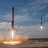1 Vegetable      309 天前 好活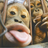2 fate      309 天前 牛皮！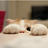3 server      309 天前4 这要是摸鱼,那我摸的啥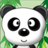4 1239305697      309 天前 能做成 emoji 吗，以后代码就用这个来写了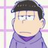5 test3207      309 天前 （ blog 证书过期啦！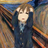6 kinglisky   OP   309 天前 @test3207 纳尼，好久没看了 🤣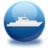7 PopRain      309 天前 训练一下 tesseract ocr，再和自己算法 PK 一下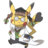8 Jrue0011      309 天前 好，就当我已经学会了9 kassadin      309 天前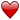10 kinglisky   OP   309 天前 @PopRain 看了看项目，嗯，是我不会的东西 😹，这块没摸过，有机会试试11 crackhopper      309 天前 已经知道了希卡文映射字母，这个先验就很强了；但还知道了图片汉明距离可以作为相似度，那就基本已经求解了。我还以为是根据字符出现的语义和场景，做统计，分词，用语言学+算法那套搞的。12 crackhopper      309 天前 哦哦，是做 OCR 。我错了。我以为是做语言识别和翻译。13 kinglisky   OP   309 天前 @crackhopper 整不了那么高深的东西，就是切割文字，然后做个图片的相似度判断，嘿嘿~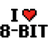14 8bit      309 天前 最喜欢这种认真搞不务正业的活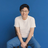15 iamsk      309 天前 给小哥点赞，编程最有趣的就是这些了👍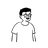16 RRRoger      309 天前 太强了17 huanghui      309 天前 via iPhone 好厉害~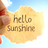18 crayygy      309 天前 via Android 🐮🍺哦，这个很强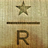19 cpstar      309 天前 额。。。我想说个啥。。。似乎这是最基本的图像识别技术，不外乎到底是什么文字，而在于原始图片库里字符和图形的对应关系。至于 OCR，那要处理的是各种变形，稍微给扭曲一下啥的，能识别。。。我能想到的就是机车号牌识别，字母数字都都有一定的标准（式样），号牌格式也有一定的标准，于是各种歪七扭八的角度，快速识别内容。20 cpstar      309 天前 至于这个希卡文，我不清楚背景是个啥，这不还是英语么，只不过弄了一对奇怪字符去对应，看似密码学，最最最最入门的那种加密方法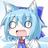21 icyalala      309 天前 @cpstar 塞尔达传说，是一个非常知名的 Switch 独占游戏。任天堂为游戏里的古代希卡族设计了一种文字，就是帖子最上方游戏截图里那种印章样的图案。单纯技术上讲可能的确没那么复杂，但是楼主做得东西很有趣~22 kinglisky   OP   309 天前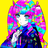23 xarthur      309 天前 这个厉害。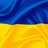24 nnikooih      309 天前 讲的蛮清楚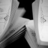25 Torpedo      309 天前 讲的挺好。关键更厉害的是有能力把这个议题搞的这么清楚。。。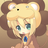26 wowboy      309 天前 哈哈哈，想起了本科室友做的车牌识别，也是用的这种方法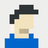27 loading      309 天前 via Android 这个和当年同学的车牌识别差不多。28 fengjianxinghun      309 天前 有点意思。正好玩塞尔达。29 whoosy      309 天前 你管这叫摸鱼？30 Lemeng      309 天前 这个真还有点意思31 SeaRecluse      309 天前 虽然很棒但是大人时代变了，传统 OCR × AI 梭哈 √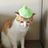32 laqow      309 天前 via Android 其实难度在算法，刨去算法剩下的框架应该都一样的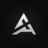33 Microi      309 天前 21 世纪社畜✘海拉鲁考古学家✓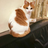34 SetsunaX      309 天前 厉害啊～35 Thymolblue      309 天前 帮大忙了！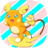36 reiji      309 天前 这个题目有意思，实现也很有启发，我有空的时候试一下用 tensorflow.js 来实现下37 HeapOverflow      309 天前 via iPhone 这摸的是鲸鱼吧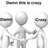38 zhutter      308 天前 硬核海拉鲁老流氓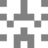39 agagega      308 天前 via iPhone 为啥比较 hash 的汉明距离就可以？两张图片如果有差，hash 不会很不一样吗？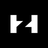40 codehz      308 天前 via Android @agagega 因为都是机器生成的，所以一定是完全一致的，这个方法就可行了。。。（甚至连投影都不需要修复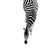41 steveway      308 天前 那是真滴 nb!